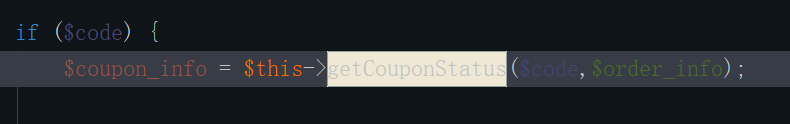# opencart关于coupon使用导致支付后订单欺诈的研究

• A+

1. public function confirm(\$order_info\$order_total) {
2.         \$code = '';
3.         \$start = strpos(\$order_total['title'], '(') + 1;
4.         \$end = strrpos(\$order_total['title'], ')');
5.         if (\$start && \$end) {
6.             \$code = substr(\$order_total['title'], \$start\$end - \$start);
7.         }
8.         if (\$code) {
9.             \$coupon_info = \$this->getCoupon(\$code);
10.             if (\$coupon_info) {
11.                 \$this->db->query("INSERT INTO `" . DB_PREFIX . "coupon_history` SET coupon_id = '" . (int)\$coupon_info['coupon_id'] . "', order_id = '" . (int)\$order_info['order_id'] . "', customer_id = '" . (int)\$order_info['customer_id'] . "', amount = '" . (float)\$order_total['value'] . "', date_added = NOW()");
12.                  /* 忽略一些自己写的SQL */
13.             } else {
14.                 return \$this->config->get('config_fraud_status_id');
15.             }
16.         }
17.     }